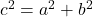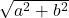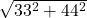## If a rectangular plot measures 33 feet by 44 feet, what is the length, in feet, of the diagonal of the plot?

Question

If a rectangular plot measures 33 feet by 44 feet, what is
the length, in feet, of the diagonal of the plot?

in progress 0
6 months 2021-07-24T11:55:18+00:00 1 Answers 5 views 0

55 feet

Step-by-step explanation:

If you draw it out, the diagnonal along wiht 2 sides of the rectangle make a rigth angles traingle.

Using pythagoras theoreom,, c is the length of the diagonal and a and b are 2 sides of rectangle.

So c==put in alculator and get:

55

=55 feet